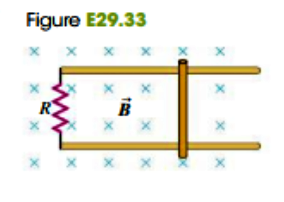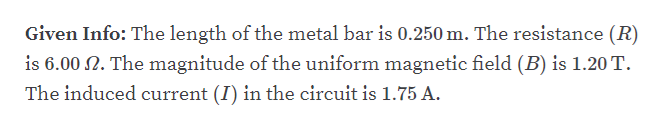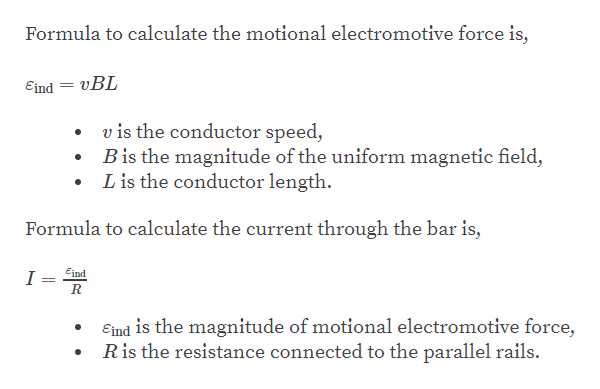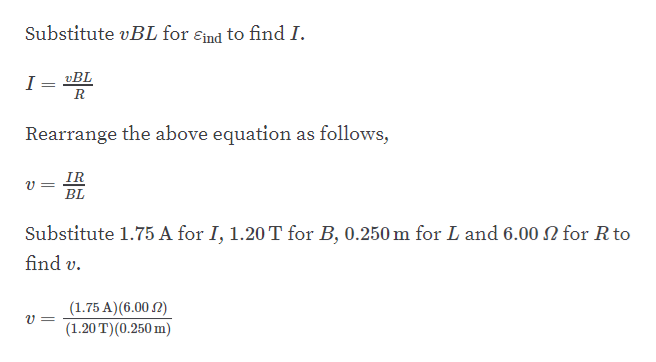Question
2 views

29.33     A 0.250-m-long bar moves on parallel rails that are connected through a 6.00-Ohm resistor, as shown in Fig. E29.33, so the apparatus makes a complete circuit.  You can ignore the resistance of the bar and rails.  The circuit is in a uniform magnetic field B = 1.20 T that is directed out of the plane of the figure.  At the instant when the induced current in the circuit is counterclockwise and equal to 1.75 A, what is the velocity of the bar (magnitude and direction)?help_outlineImage TranscriptioncloseFigure E29.33 х х х в х х х х fullscreen
check_circle

Step 1help_outlineImage TranscriptioncloseGiven Info: The length of the metal bar is 0.250 m. The resistance (R) is 6.00 2. The magnitude of the uniform magnetic field (B) is 1.20 T. The induced current (I) in the circuit is 1.75 A. fullscreen
Step 2help_outlineImage TranscriptioncloseFormula to calculate the motional electromotive force is, Eind = vBL v is the conductor speed, Bis the magnitude of the uniform magnetic field, L is the conductor length Formula to calculate the current through the bar is, I = ad Eind is the magnitude of motional electromotive force, Ris the resistance connected to the parallel rails. fullscreen
Step 3help_outlineImage TranscriptioncloseSubstitute vBL for End to find I. I = »BL Rearrange the above equation as follows, IR BL Substitute 1.75 A for I, 1.20 T for B, 0.250 m for L and 6.00 2 for R to find v (1.75 A) (6.00 2) |(1.20 T)(0.250 m) fullscreen

### Want to see the full answer?

See Solution

#### Want to see this answer and more?

Solutions are written by subject experts who are available 24/7. Questions are typically answered within 1 hour.*

See Solution
*Response times may vary by subject and question.
Tagged in

### Current Electricity